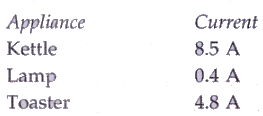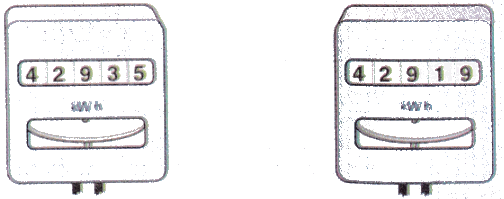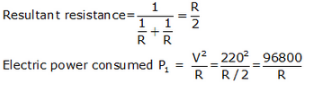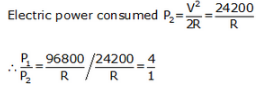Courses

# Solutions of Electricity (Page No- 60) - Physics By Lakhmir Singh, Class 10 Class 10 Notes | EduRev

## Class 10 : Solutions of Electricity (Page No- 60) - Physics By Lakhmir Singh, Class 10 Class 10 Notes | EduRev

The document Solutions of Electricity (Page No- 60) - Physics By Lakhmir Singh, Class 10 Class 10 Notes | EduRev is a part of the Class 10 Course Class 10 Physics Solutions By Lakhmir Singh & Manjit Kaur.
All you need of Class 10 at this link: Class 10

Lakhmir Singh Physics Class 10 Solutions Page No:60

Question 41:
State whether an electric heater will consume more electrical energy or less electrical energy per second when the length of its heating element is reduced. Give reasons for your answer.
Solution :
By reducing the length of element the resistance will decrease.
Power is inversely proportional to resistance. So, this will result in more consumption of energy.

Question 42:
The table below shows the current in three different electrical appliances when connected to the 240 V mains supply :(a) Which appliance has the greatest electrical resistance ? How does the data show this ?
(b) The lamp is connected to the mains supply by using a thin, twin-cored cable consisting of live and neutral wires. State two reasons why this cable should not be used for connecting the kettle to the mains supply.
(c) Calculate the power rating of the kettle when it is operated from the 240 V mains supply.
(d) A man takes the kettle abroad where the mains supply is 120 V. What is the current in the kettle when it is operated from the 120 V supply ?

Solution :
(a) Lamp; because least current is flowing through it.
(b) Large current drawn by the kettle; Earth connection needed.
(c) We know that
P = VI
V= 240V, I = 8.5A
P = 240X8.5 = 2040W = 2.04kW
(d) When connected to 240 V supply, P = 2040W
R = V2/P = 2402/240
R = 28.23ohm
Now, when V = 120V, R = 28.23ohm
I = V/R = 120/28.23 = 4.25A

Question 43:
A boy noted the readings on his home’s electricity meter on Sunday at 8 AM and again on Monday at 8 AM (see figure below).(a) What was the meter reading on Sunday ?
(b) What was the meter reading on Monday ?
(c) How many units of electricity have been used ?
(d) In how much time these units have been used ?
(e) If the rate is Rs. 5 per unit, what is the cost of electricity used during this time ?

Solution :
(a) 42919
(b) 42935
(c) 42935-42919=16 units
(d) 24 hours
(e) Cost of 1 unit = Rs. 5
Cost of 16 units = 16×5 = Rs. 80

Question 44:
An electric bulb is rated as 10 W, 220 V. How many of these bulbs can be connected in parallel across the two wires of 220 V supply line if the maximum current which can be drawn is 5 A ?

Solution :
P = 10W, V = 220V, I = 5A
We know that
P = VI
= 220X5
P = 1100W
Power of one bulb = 10W
Total no. of bulbs that can be connected = 1100/10 = 110

Question 45:
Two exactly similar electric lamps are arranged (i) in parallel, and (ii) in series. If the parallel and series combination of lamps are connected to 220 V supply line one by one, what will be the ratio of electric power consumed by them ?

Solution :

Let resistance of each lamp = R ohms.
Case 1: Parallel connectionCase 2: Series connection
Resultant resistance = R+R = 2ROffer running on EduRev: Apply code STAYHOME200 to get INR 200 off on our premium plan EduRev Infinity!

94 docs

,

,

,

,

,

,

,

,

,

,

,

,

,

,

,

,

,

,

,

,

,

,

,

,

;